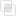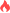python复合赋值运算符是什么2021-06-29 14:44:40464浏览 · 0收藏 · 0评论1、当解释器执行到复合赋值运算符时，先计算算数运算符的表达式，再将算数运算符执行后的结果赋值到等号左边的变量。

2、复合赋值可以让程序更加精炼，提高效率。

+=       加法赋值运算符

-=       减法赋值运算符

*=        乘法赋值运算符

/=        除法赋值运算符

```# 输出101  a = a + 1,最终a = 100 + 1
a = 100
a += 1
print(a) # 101

# 输出6  b = b * 3,最终b = 2 * 3
b = 2
b *= 3
print(b)

# 输出13, 先算运算符右侧1 + 2 = 3， c += 3 , 推导出c = 10 + 3
c = 10
c += 1 + 2
print(c)
# 注意： 先算复合赋值运算符右面的表达式，再算复合赋值运算.
d = 100
d *= 3 + 4
print(d) # 700
# 3 + 4 = 7 * 100 = 700```python中Pylint是什么python中Pylint的信息类型python如何封装特性python继承的特性分析python多态的开闭原则python return和yield的执行比较python如何用函数创造字典python中ThreadPoolExecutor如何使用python单分支结构是什么python二分支结构如何理解python多分支结构是什么python小整数池是什么python inter机制是什么意思python表达式是什么php设置SESSION_ID的方法578

php中nts是什么308

JavaScript处理并行请求的方法192